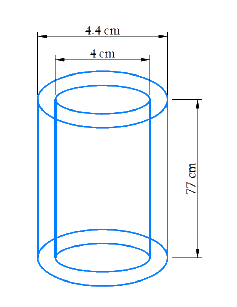# Ex.13.2 Q3 Surface Areas and Volumes - NCERT Maths Class 9

## Question

A metal pipe is $$77\,\rm{ cm}$$ long. The inner diameter of a cross section is $$4\,\rm{ cm},$$ the outer diameter being $$4.4\,\rm{ cm}$$ . Find it

(i) Inner curved surface area

(ii) Outer curved surface area

(iii) Total surface area.

Video Solution
Surface Areas And Volumes
Ex 13.2 | Question 3

## Text Solution

Reasoning:

The curved surface area of a right circular cylinder of base radius $$r$$  and height is $$\rm 2 \pi rh$$ . And its total surface area is \begin{align}2\pi r(r + h) \end{align}.

What is the known?

Length of the metal pipe, the inner and outer diameter of the cross sector of the metal pipe.

(i) Inner curved surface area

Steps:

\begin{align}{\rm{height}}(h) &= 77\,\,\rm{cm}\\{\rm{diameter} }&= 2r = 4\,\,\rm{cm}\\r &= 2\,\,\rm{cm} \end{align}

Inner curved surface area
\begin{align}&= 2\pi rh\\ &= 2 \times \frac{{22}}{7} \times 2 \times 77\\ &= 968\,\,\rm{c{m^2} } \end{align}

(ii) Outer curved surface area

Steps:

\begin{align}{\rm{{height}}} (h) &= 77\,\,\rm{cm}\\\rm{diameter} &= 2R = 4.4\,\,\rm{cm}\\R &= 2.2\,\,\rm{cm} \end{align}

Inner curved surface area

\begin{align}&= 2\pi Rh\\ &= 2 \times \frac{{22}}{7} \times 2.2 \times 77\\ &= 1064.8\,\,\rm{c{m^2}} \end{align}

(iii) Total surface area

Steps:

Total surface area of pipe = Curved Surface Area of inner surface + Curved Surface Area of outer surface + Area of both the circular ends of the pipe

Total surface area

\begin{align} &= \,\,2\pi Rh + 2\pi rh + 2\pi ({R^2} - {r^2}]\\ &= 1064.8 \!+\! 968 \!+\! 2\! \times \!\frac{{22}}{7} \!\times\! [{(2.2)^2}\! - \!{(2)^2}]\\ &= 1064.8 + 968 + 5.28\\ &= 2038.08\,\,\,\rm{c{m^2}} \end{align}Inner curved surface area \begin{align} = 968\,\,\rm{c{m^2}} \end{align}

Outer curved surface area \begin{align} = 1064.8\,\,\rm{c{m^2}} \end{align}

Total surface area \begin{align} = 2038.08\,\,\rm{c{m^2}} \end{align}

Learn from the best math teachers and top your exams

• Live one on one classroom and doubt clearing
• Practice worksheets in and after class for conceptual clarity
• Personalized curriculum to keep up with school